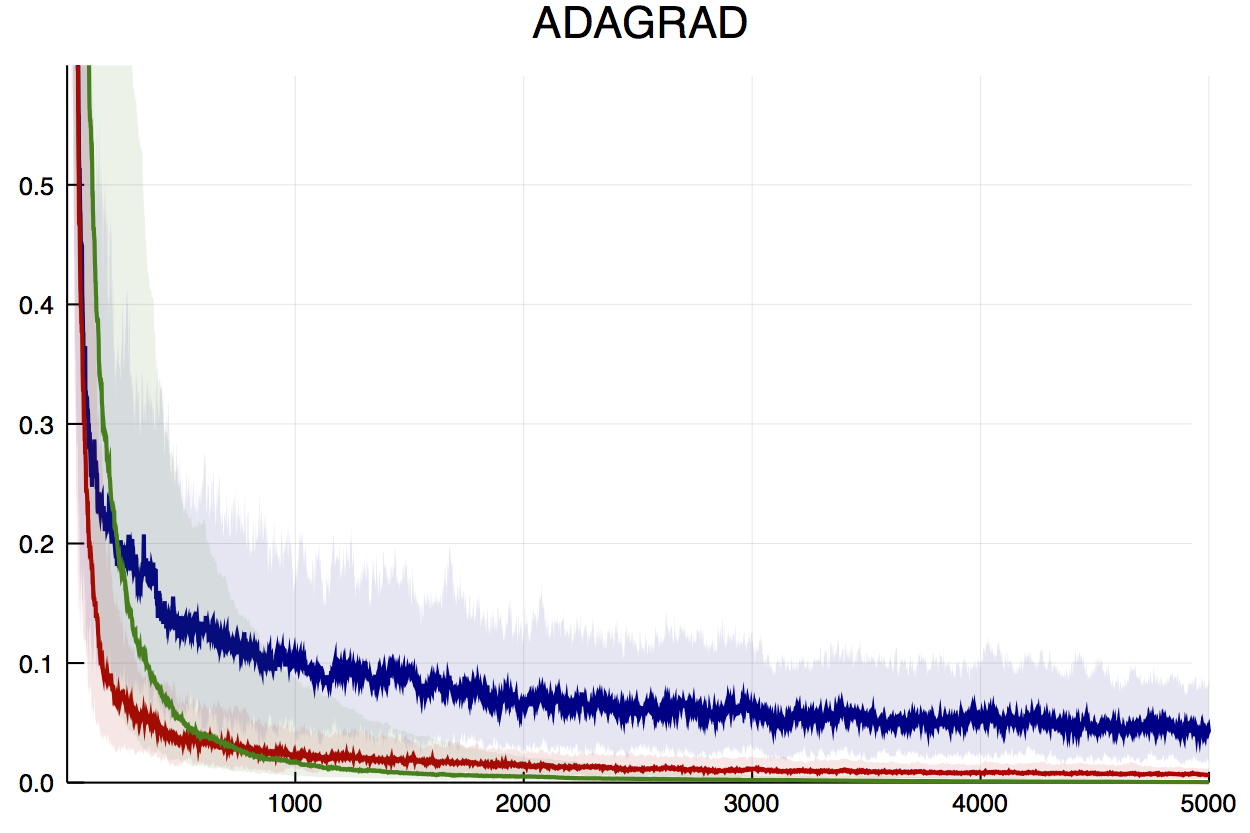# Adagrad Has a Learning Rate

AdaGrad (PDF) is an interesting algorithm for adaptive element-wise learning rates. First consider a stochastic gradient descent (SGD) update

$$\theta^{(t)} = \theta^{(t-1)} + \gamma_t g_t,$$

where $\gamma_t$ is a step size (learning rate) and $g_t = \nabla f_t(\theta^{(t-1)})$ is the gradient of a noisy objective function. The AdaGrad algorithm replaces the step sizes $\gamma_t$ with a matrix inverse that scales the elements of the gradient:

$$\theta^{(t)} = \theta^{(t-1)} + \text{diag}(G_t)^{-\frac{1}{2}}g_t,$$

where $Gt = \sum{i=1}^t g_t g_t^T$. In this form, it is suggested that AdaGrad does not have a learning rate. However, with very basic algebra, the AdaGrad update is

$$\theta^{(t)} = \theta^{(t-1)} + \frac{1}{\sqrt t}\text{diag}(G_t^*)^{-\frac{1}{2}}g_t,$$

where $G_t^* = t^{-1} G_t$. That is, if the matrix term producing the adaptive step sizes is considered as a mean instead of a sum, we see that AdaGrad has a learning rate $\gamma_t = 1/\sqrt t$.

## OnlineStats

In OnlineStats.jl, different weighting schemes can be plugged into any statistic or model (see docs on Weights). OnlineStats implements AdaGrad in the mean form, which opens up a variety of learning rates to try rather than just the default $\gamma_t = \frac{1}{\sqrt{t}}$. The plot below compares three different choices of learning rate. It’s interesting to note that the blue line, which uses the above default, is the slowest at finding the optimum loss value.To try out OnlineStats.ADAGRAD, see StatLearn